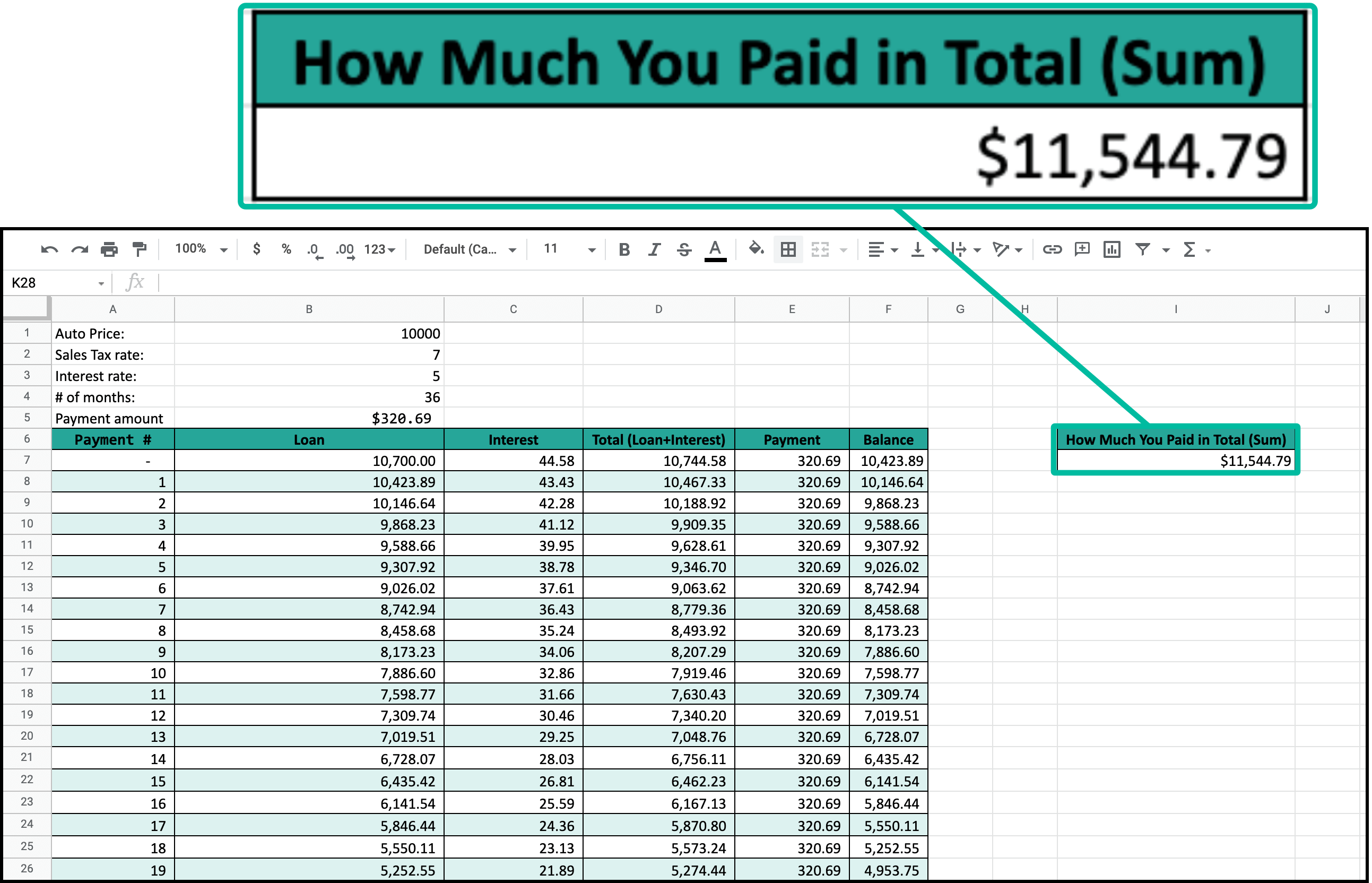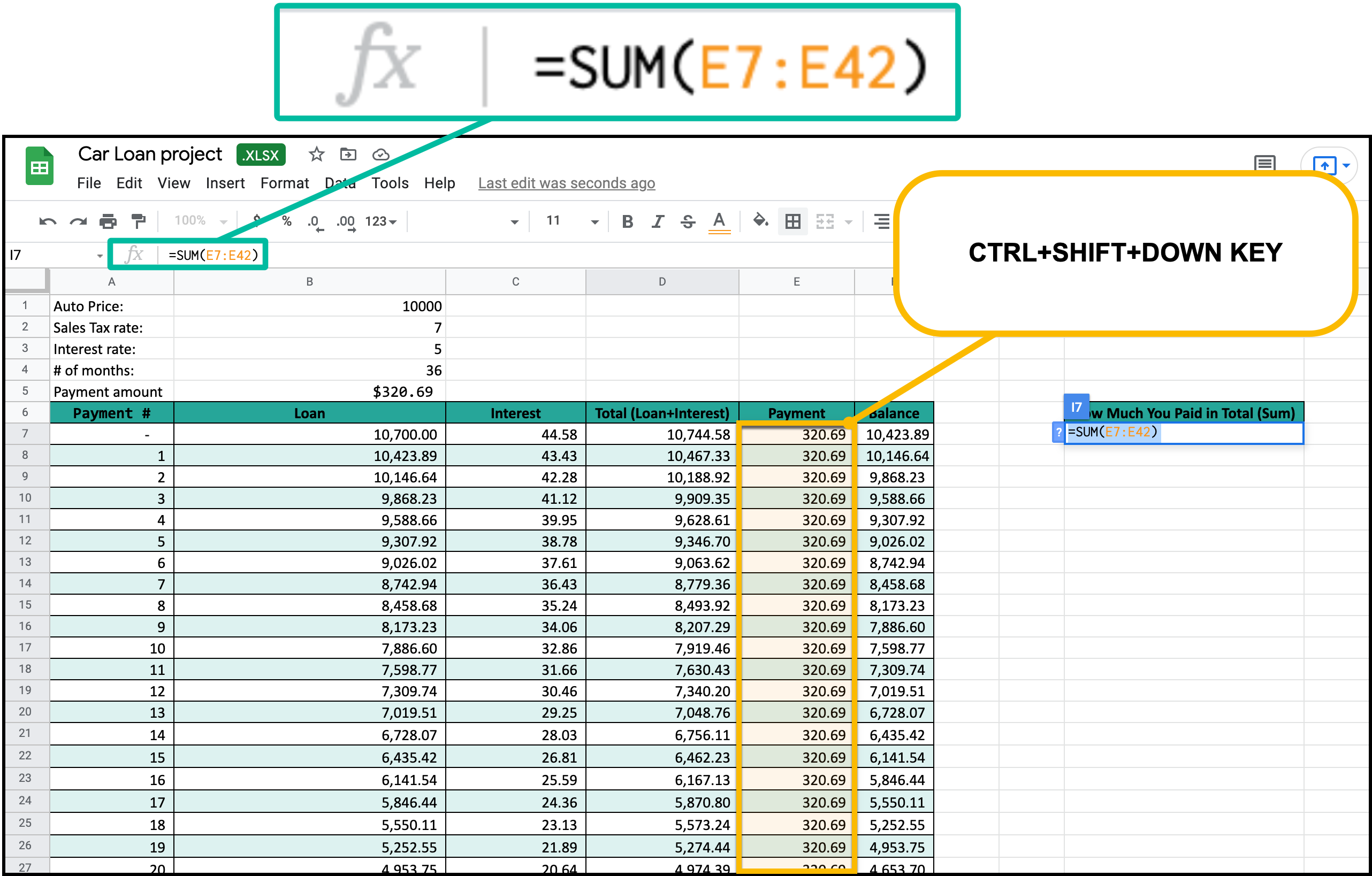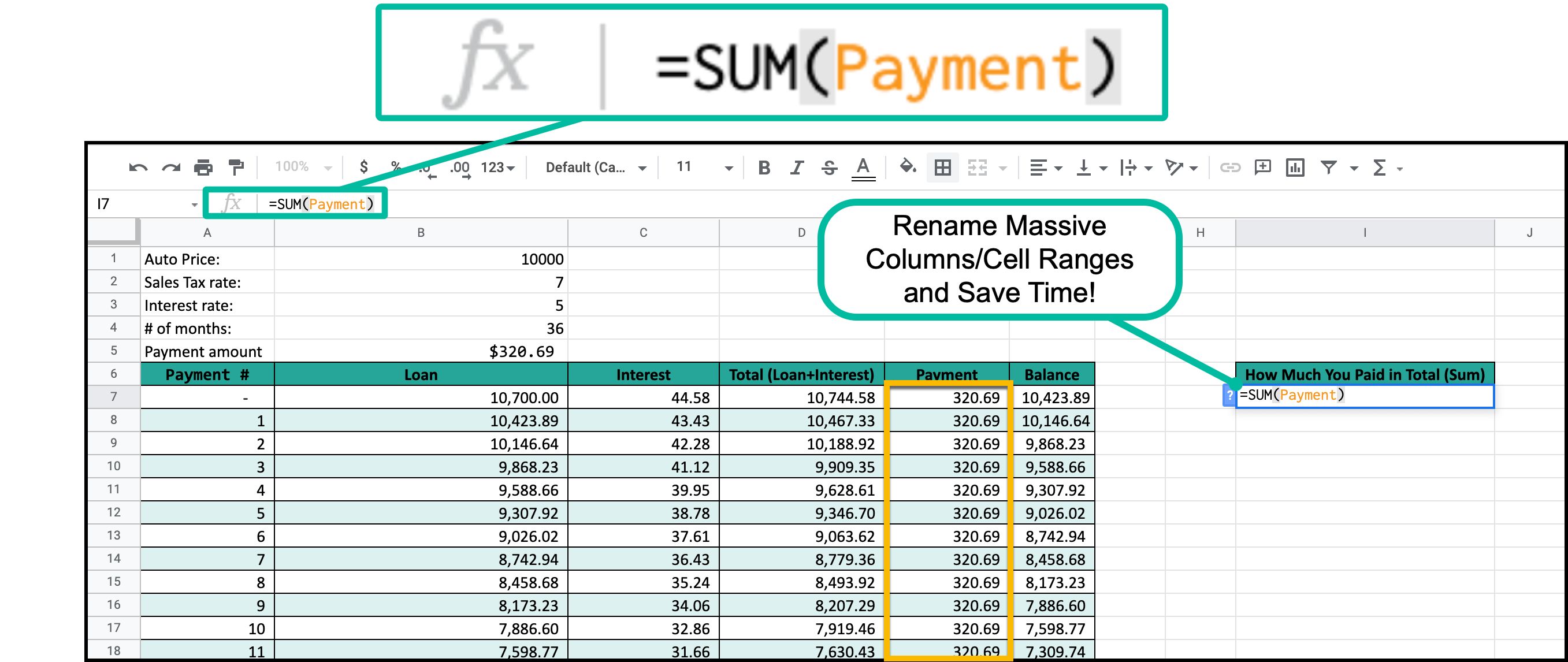# How to Find the Sum of a Column in Google SheetsUse this technique to quickly find the sum of an entire column of data in Google Sheets quickly and effectively.

Without further ado, let's get right into it

## =SUM(Column Cell Range)To fill in the function as quickly as possible you should follow these steps:

1. Select where you want to place the sum: I like to put my sum and calculation cells away from the actual data table.
2. Use the "=SUM()" Formula: there is another post dedicated to understanding the SUM function.
3. Reference the column: What I do is I like to do is click the first cell of teh column I want to sum and then I use the Keyboard Shortcut CRTL+SHIFT+DOWN. That will automatically highlight every cell for you. Alternatively, you could also name the cell range ahead of time (which is what I like to do) and save yourself the hassle in the moment.Upon entering the formula, you should be provided with a sum of every cell in your desired column.

And there you have it; that is how you find the sum of an entire column within Google Sheets!